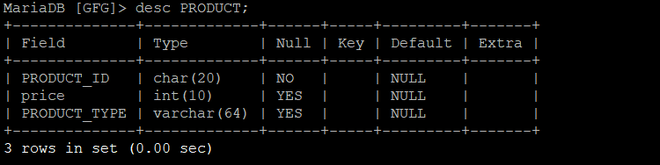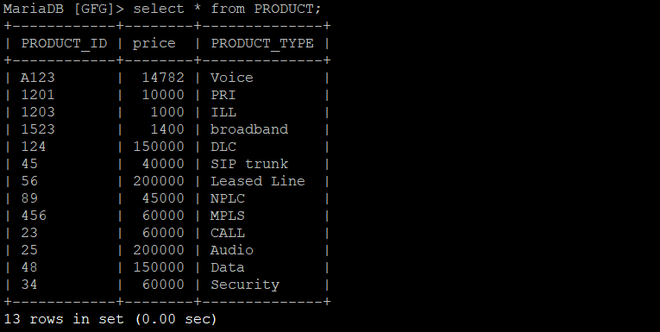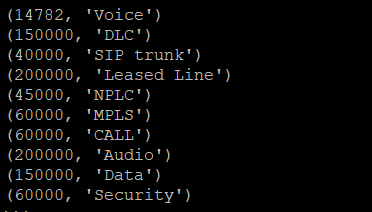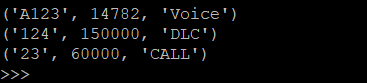Open in App
Not now

# Python MariaDB – Where Clause using PyMySQL

• Last Updated : 14 Oct, 2020

Where clause is used in MariaDB database to filter the data as per the condition required. You can fetch, delete or update a particular set of data in MariaDB database by using the where clause.

Syntax :

```SELECT column1, column2, …. cloumnN FROM [TABLE NAME] WHERE [CONDITION];

```

The above syntax is used for displaying a certain set of data following the condition.

Example 1: Consider the following database named GFG and having a table name as a PRODUCT.

Schema of the Table:Table data :Where Clause In Python :

Steps to use where clause in Python is:

1. First form a connection between MariaDB and Python program. It is done by importing pymysql package and using pymysql.connect() method, for passing the username, password, host (optional default: localhost) and, database (optional) as parameters to it.
2. Now, create a cursor object on the connection object created above by using the cursor() method. A database cursor is a control structure that enables traversal over the records in a database.
3. Then, execute the where clause statement by passing it through the execute() method.

## Python3

 `import` `pymysql`` ` `# Create a connection object``# IP address of the MySQL database server``Host ``=` `"localhost"`  ` ` `# User name of the database server``User ``=` `"user"`       ` ` `# Password for the database user``Password ``=` `""           `` ` `database ``=` `"GFG"`` ` `conn  ``=` `pymysql.connect(host``=``Host, user``=``User, password``=``Password, database)`` ` `# Create a cursor object``cur  ``=` `conn.cursor()`` ` ` ` `query ``=` `f``"SELECT price,PRODUCT_TYPE FROM PRODUCT WHERE price > 10000"`` ` `cur.execute(query)`` ` `rows ``=` `cur.fetchall()``for` `row ``in` `rows :``    ``print``(row)`` ` `conn.close()`

Output :Example 2 :

## Python3

 `import` `pymysql`` ` `# Create a connection object`` ` `conn  ``=` `pymysql.connect(``'localhost'``, ``'user'``, ``'password'``, ``'database'``)`` ` `# Create a cursor object``cur  ``=` `conn.cursor()`` ` ` ` `query ``=` `f``"SELECT * FROM PRODUCT WHERE PRODUCT_TYPE in ('Voice','DLC','CALL')"`` ` `cur.execute(query)`` ` `rows ``=` `cur.fetchall()``for` `row ``in` `rows :``    ``print``(row)`` ` `conn.close()`

Output :My Personal Notes arrow_drop_up